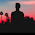# Refraction through a Prism Viva Questions

The Refraction through a Prism Viva Questions

(i) What is meant by refractive index of the material of a prism?

Ans: It is the ratio of the velocity of light in free space to the velocity of light in the material

(ii) What is the relation between angle of incidence and angle of emergence, when the angle of deviation is minimum?

Ans: The angle of incidence and angle of emergence are equal. The ray passes symmetrically through the prism.

(iii) Does the angle of minimum deviation depends on the colour of light?

Ans: Yes

(iv) What is critical angle of a medium?

Ans: It is the angle of incidence in the medium for which angle of refraction in air is 90°

(v) What is the relation between refractive index (n) and critical angle C?

Ans: n = 1/ sin C.

(vi) What is the unit of refractive index?

Ans: Since it is a ratio, it has no unit

(vii) What is total internal reflection?

Ans: If the angle of incidence in a denser medium is greater than the critical angle the ray is reflected internally. This is known as total internal reflection.

(viii) Aim of refraction through a prism experiment

(i) To find the angle of a prism (A)
(ii) To study the variation of the angle of deviation (d) with the angle of incidence (i) and hence to determine the angle of minimum deviation of the prism by drawing i – d curve.
(iii) To calculate the refractive index of the material of the prism.

(ix) Apparatus of refraction through a prism experiment

Prism, pins, drawing board, drawing paper, etc

(x) Theory of refraction through a prism experiment

The refractive index of the material of the prism is given by, μ = [sin(A + D)/2]/ sin A/2; where A is the angle and D is the angle of minimum deviation of the prism.

(xi) Procedure to find the angle of the prism (A)
A drawing paper is fixed on a horizontal drawing board. The outline ABC of the prism is drawn on the paper. The prism is removed. Two parallel lines are drawn at a convenient distance apart to meet the refracting faces AB and AC of the prism. Two pins P1 and Q1 are fixed on the line drawn to the face AB. The prism is placed back to its position. Two other pins R1 and S1 are fixed so that they are in line with the reflected images of P1 and Q1 at the face AB. Similarly two pins P2 and Q2 are fixed on the other line. The pins R2 and S2 are fixed so that they are in line with the reflected images of P2 and Q2 at the face AC. The prism and the pins are removed. The reflected rays R1 S1 and R2 S2 are drawn to meet at O. The angle S1 O S2 is measured. It gives 2A, twice the angle of the prism. From this A, the angle of the prism is found out. The experiment is repeated and the mean angle A of the prism is determined.

(xii) Procedure to determine the angle of minimum deviation D
A drawing paper is fixed on a horizontal drawing board. The prism is placed on it and its outline ABC is traced on the paper. AB and AC are the refracting faces and BC is the base of the prism. The prism, is removed. At a convenient point on AB a normal NN' is drawn to AB. A line PQ is drawn making an angle i, say, 30o with the normal. Two pins P1 and P2 are fixed on this line. The prism is placed on the paper at its position. Looking through the face AC two other pins P1’; and P2’ are fixed in line with images of P1 and P2. The prism and pins are removed from the paper. A straight line RS is drawn passing, through the positions of the pins P1’ and P2’. Then RS is the emergent ray corresponding to the incident ray PQ. The incident ray PQ and the emergent ray RS are produced to meet at O. The angle of deviation d is measured. The experiment is repeated for angles of incidence 35°, 40°, ... 60° and the corresponding angles of deviation are measured. A graph is drawn with i along the X-axis and d along the Y-axis. The deviation at the lowest point on the graph gives the angle of minimum deviation D.

(xiii) Procedure to calculate the refractive index of the material of the prism

The angle A and the angle of minimum deviation D of the prism are determined as explained above. The refractive index of the material of the prism is calculated using the relation,
N = [sin(A + D)/2] /sin A/2

##### 4 on: "Refraction through a Prism Viva Questions"
1.2.can't we find the angle of prism simply by protractor?

3.very useful

4.Very simple tq sir